Next: MINIMUM EXACT COVER Up: Covering, Hitting, and Splitting Previous: MAXIMUM SET SPLITTING   Index

### MINIMUM SET COVER

• INSTANCE: Collection C of subsets of a finite set S.
• SOLUTION: A set cover for S, i.e., a subset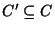such that every element in S belongs to at least one member of C'.
• MEASURE: Cardinality of the set cover, i.e.,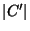.

• Good News: Approximable within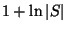.
• Bad News: Not approximable within, for some c > 0 .
• Comment: Approximable within. Not approximable withinfor any, unless NP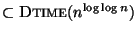. Equivalent to MINIMUM DOMINATING SET under L-reduction and equivalent to MINIMUM HITTING SET . If it is NP-hard to approximate within, then it is complete for the class of-approximable problems . Approximable within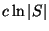if every element of S belongs to at least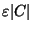sets from C for any c>0 andand . Variation in which the subsets have positive weights and the objective is to minimize the sum of the weights in a set cover is also approximable within. The problem MINIMUM K-SET COVER, the variation in which the cardinality of all sets in C are bounded from above by a constant k is APX-complete and is approximable within. Variation in which also the number of occurrences in C of any element is bounded by a constant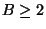is APX-complete  and approximable within B even for the general weighted problem  and . If, moreover, each element needs to be covered by at least K sets with K constant, this variation is approximable within B-K+1 . Approximable within 2 if the set system (S,C) is tree representable . The maximum coverage problem, where the input is extended with an integer k and we seek to maximize the number of covered elements using at most k sets, is approximable within e/(e-1)  and not within e/(e-1) - o(1) . The constrained variation in which the input is extended with a positive integer k and a subset T of C, and the problem is to find the set cover of size k that contains the largest number of subsets from T, is not approximable withinfor some. Variation in which a distance matrix between pairs of elements in S is given and the measure of the solution is not the cardinality of the cover but its diameter (i.e., the maximum distance of any pair of elements in C') is not approximable within any constant in the general case. If the distance matrix satisfies the triangle inequality then this variation can be approximated within 2 and no better approximation is possible. Similar results hold if the cover can be partitioned into clusters and the goal is to minimize the maximum diameter over all clusters  (see also MINIMUM K-CLUSTERING).
• Garey and Johnson: SP5Next: MINIMUM EXACT COVER Up: Covering, Hitting, and Splitting Previous: MAXIMUM SET SPLITTING   Index
Viggo Kann
2000-03-20